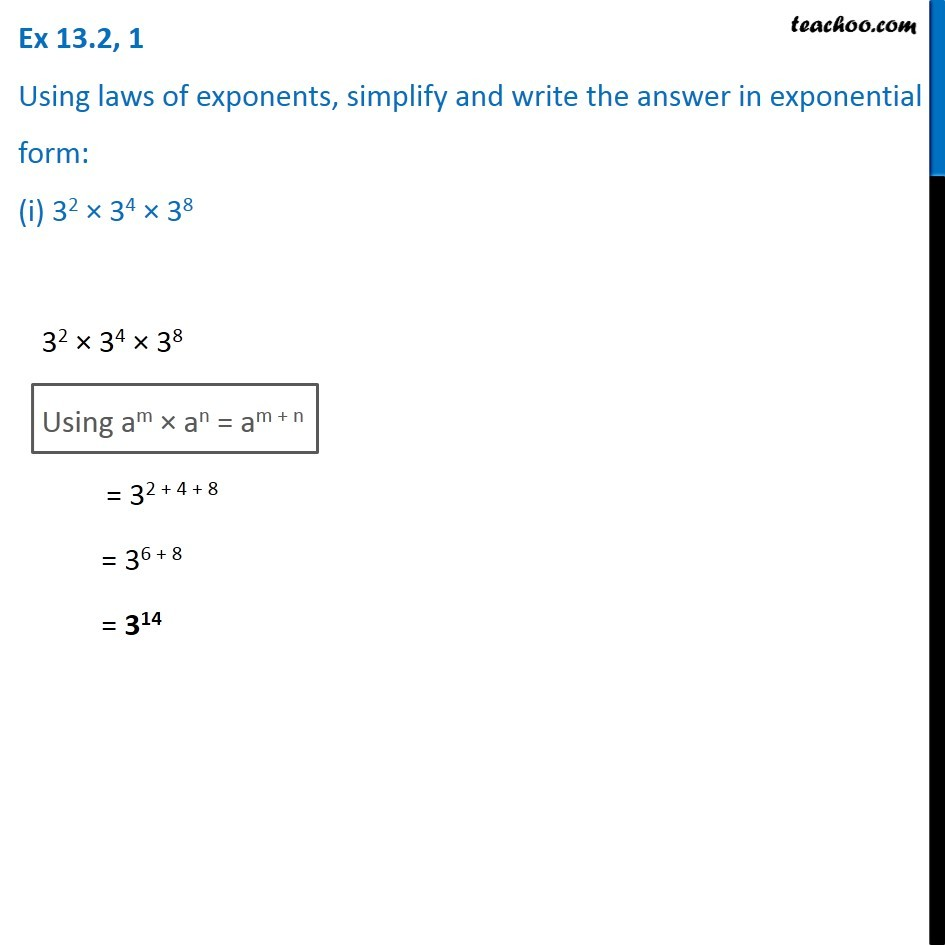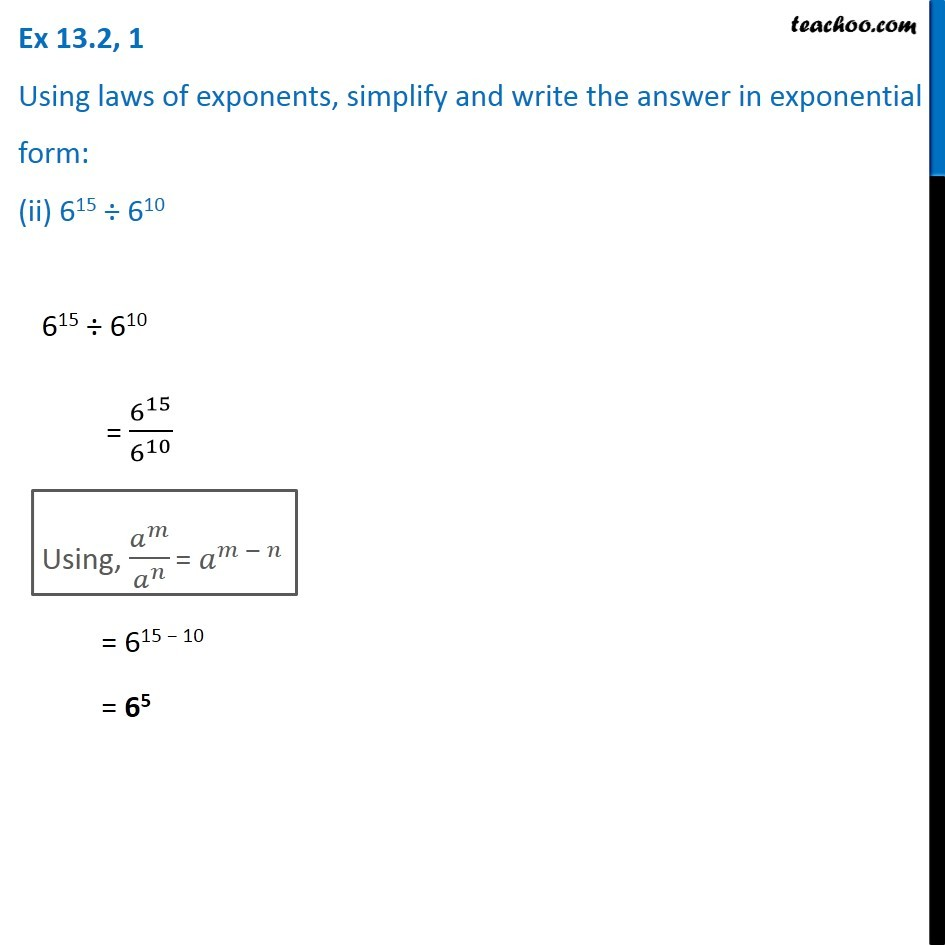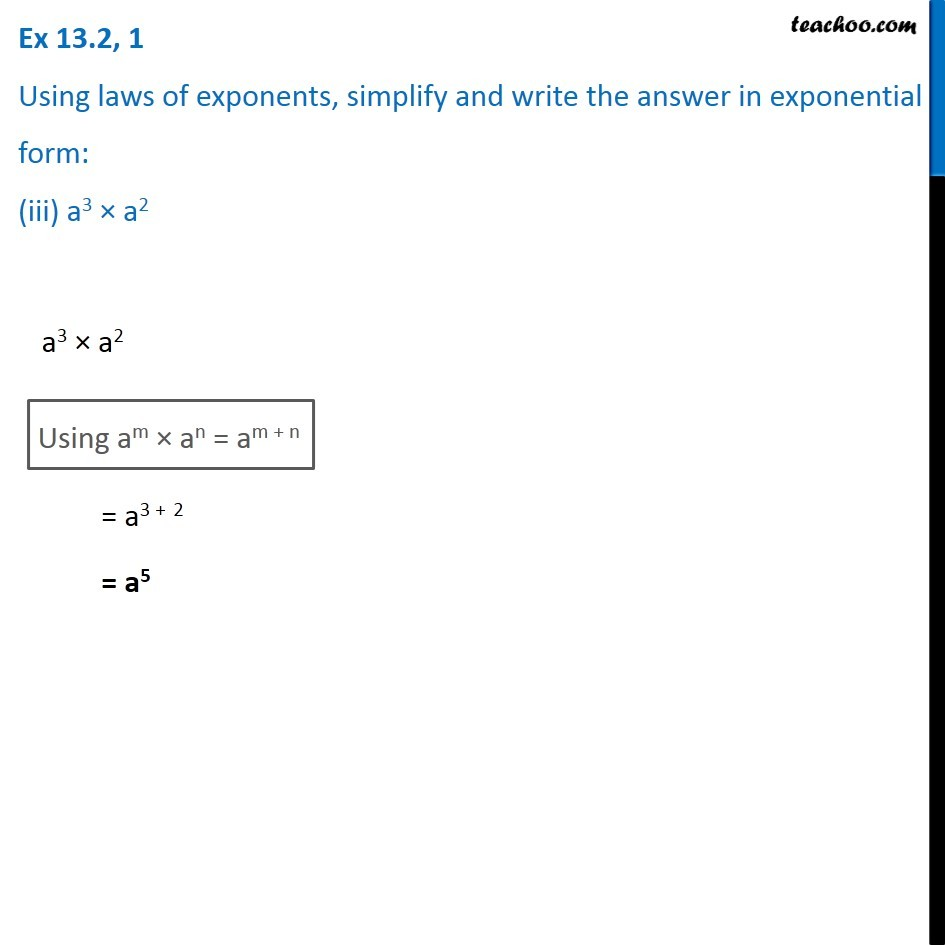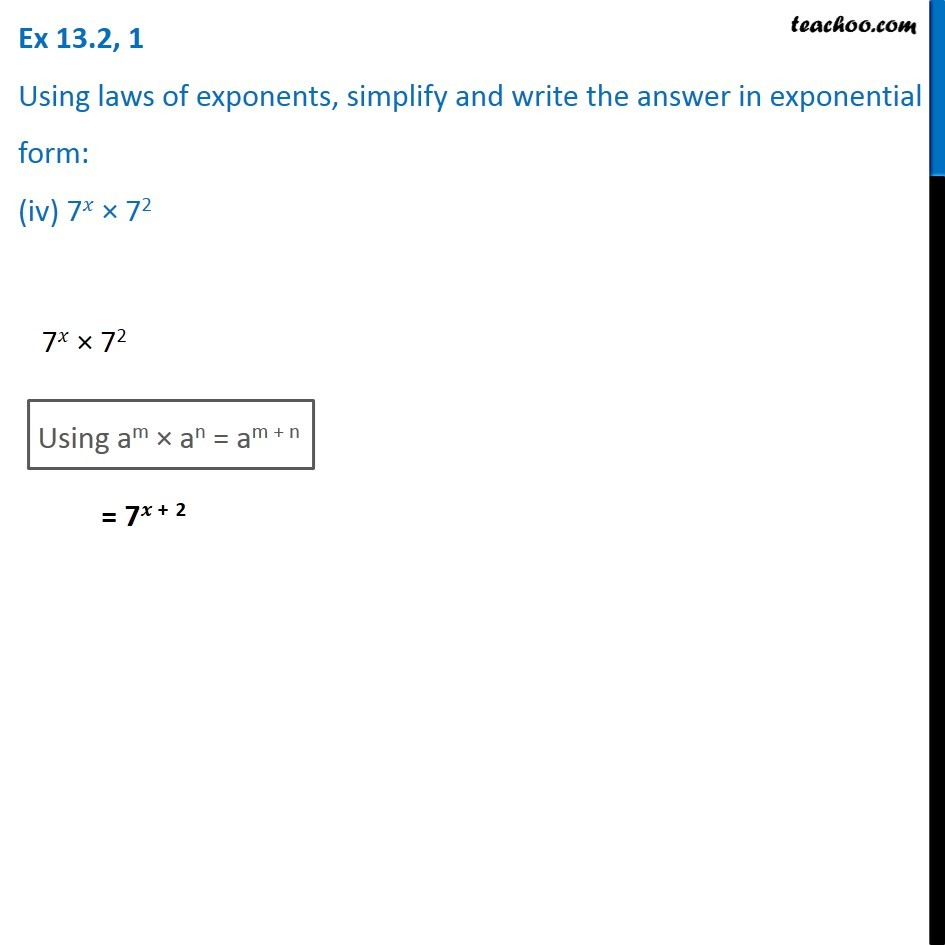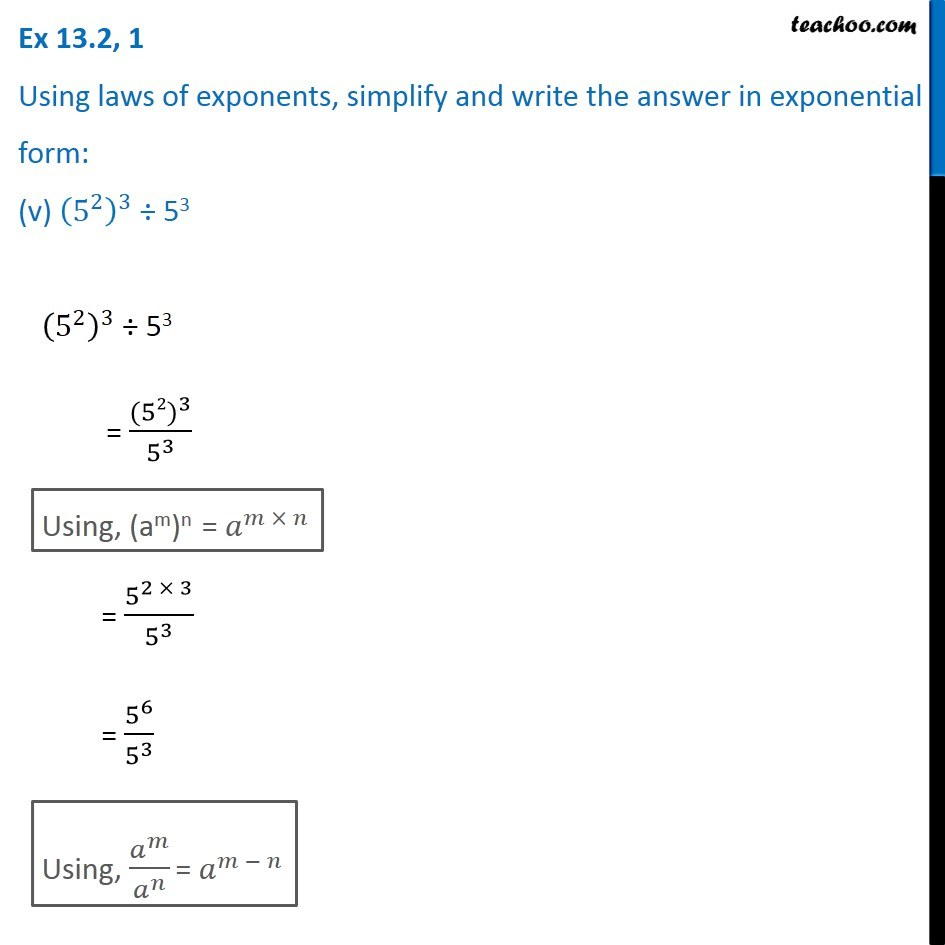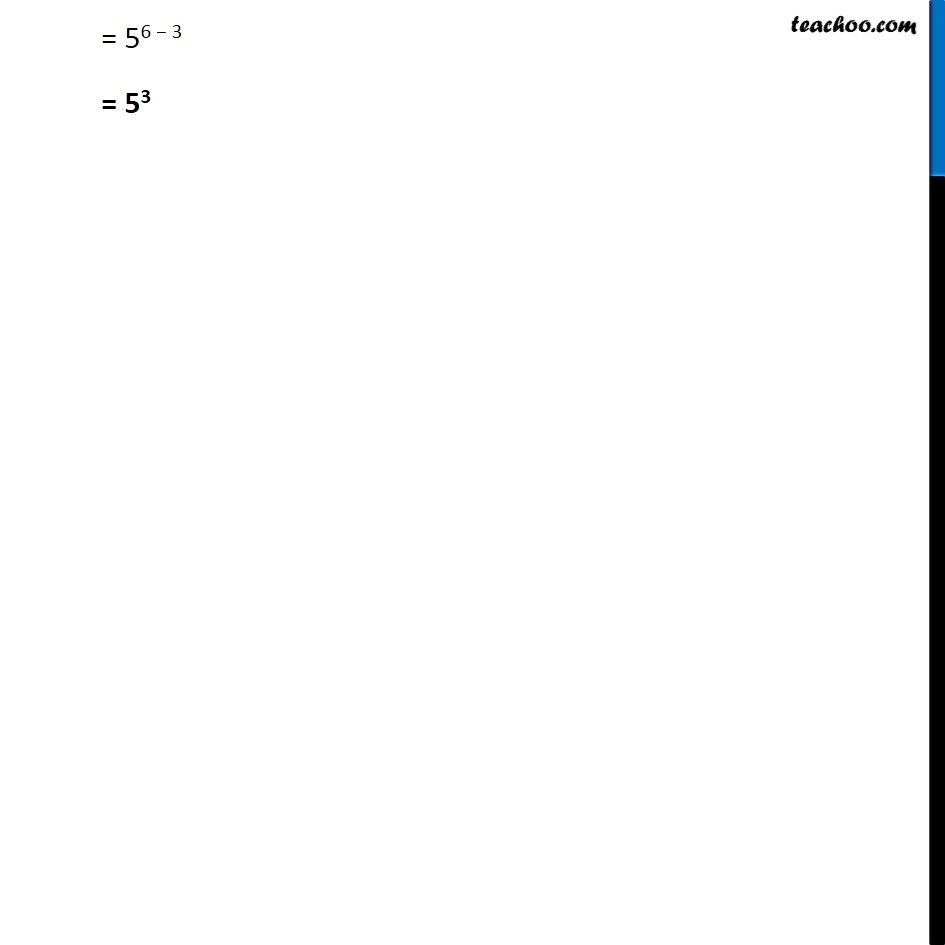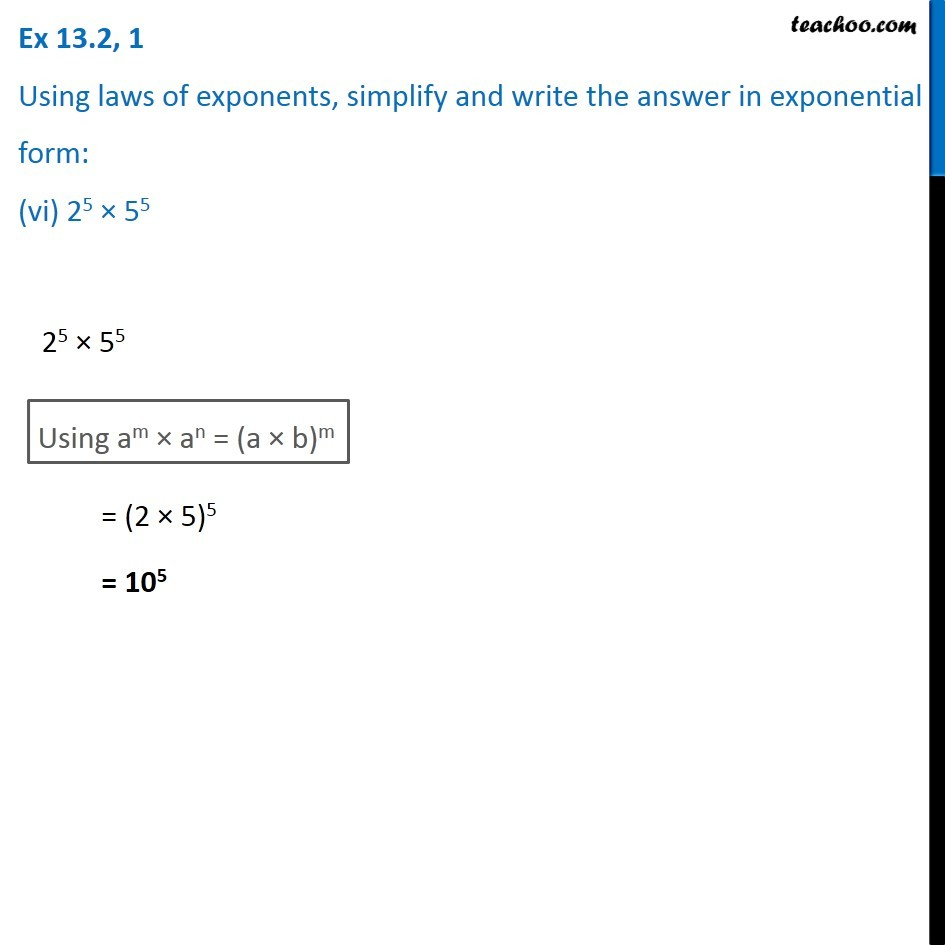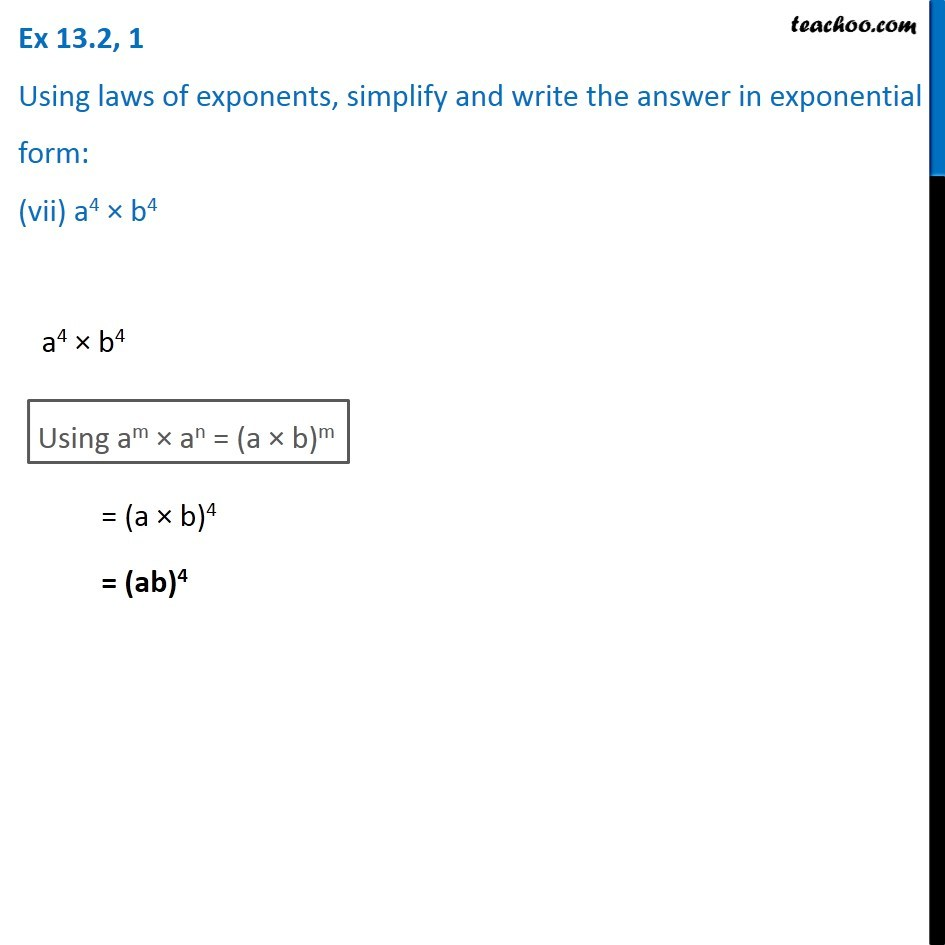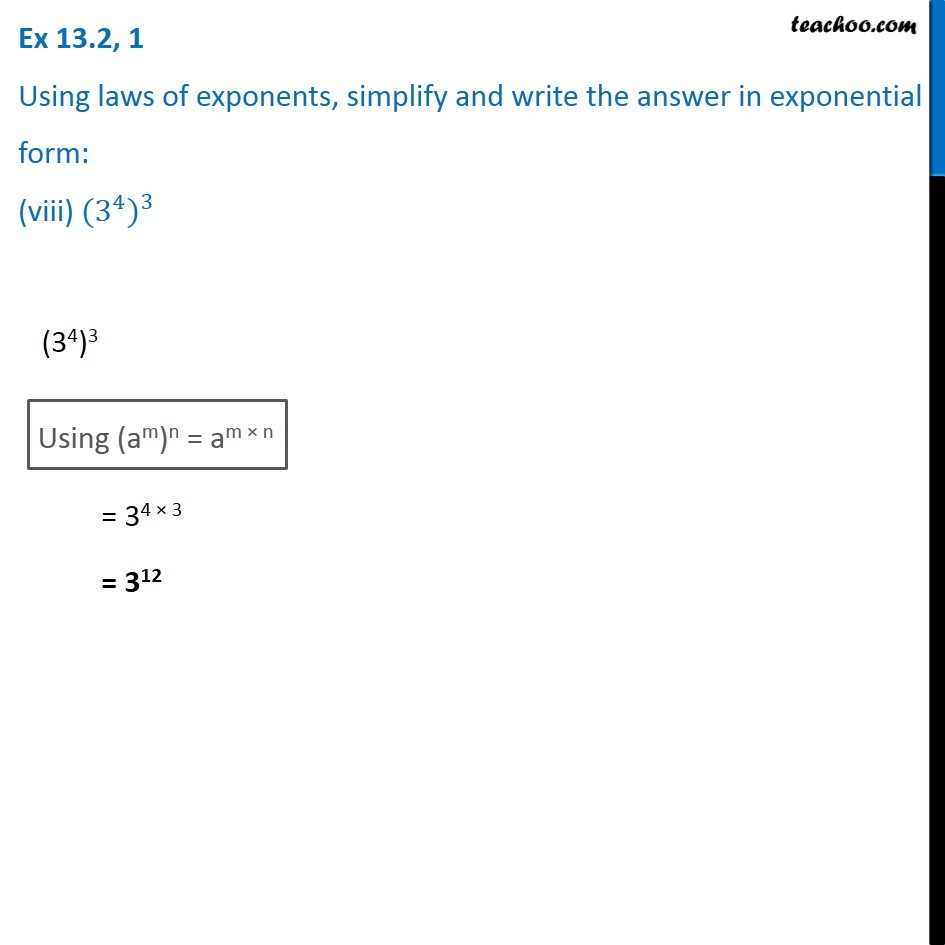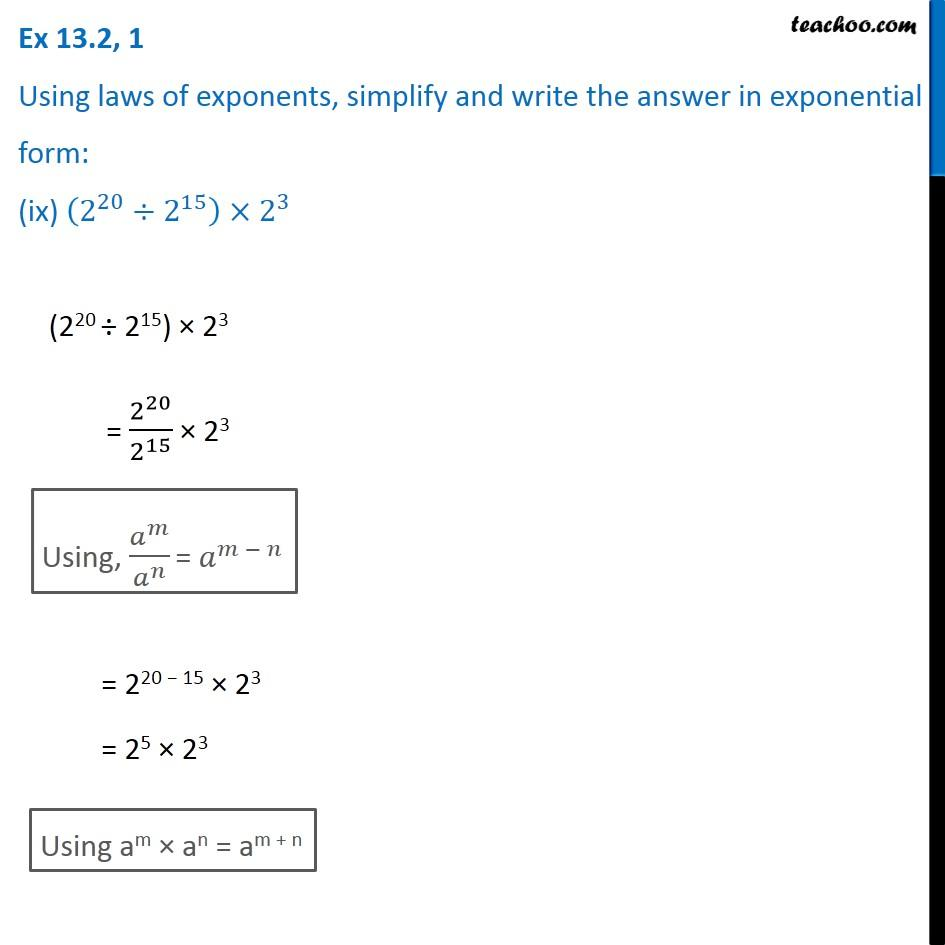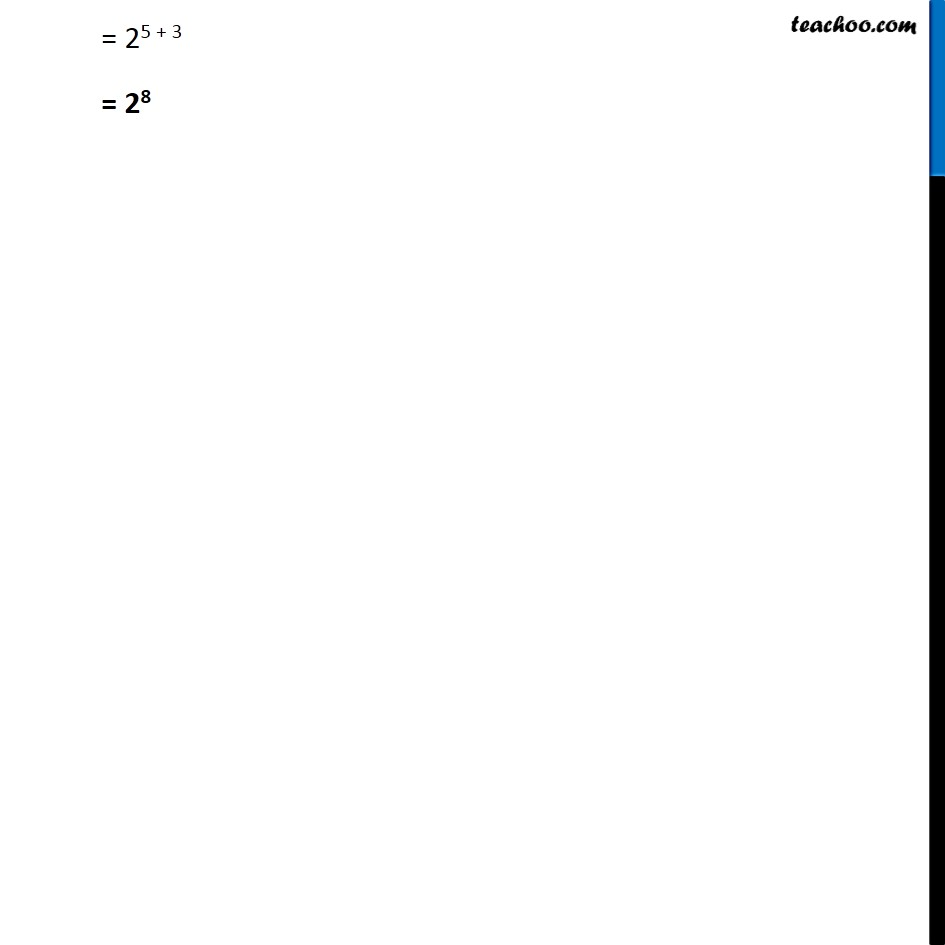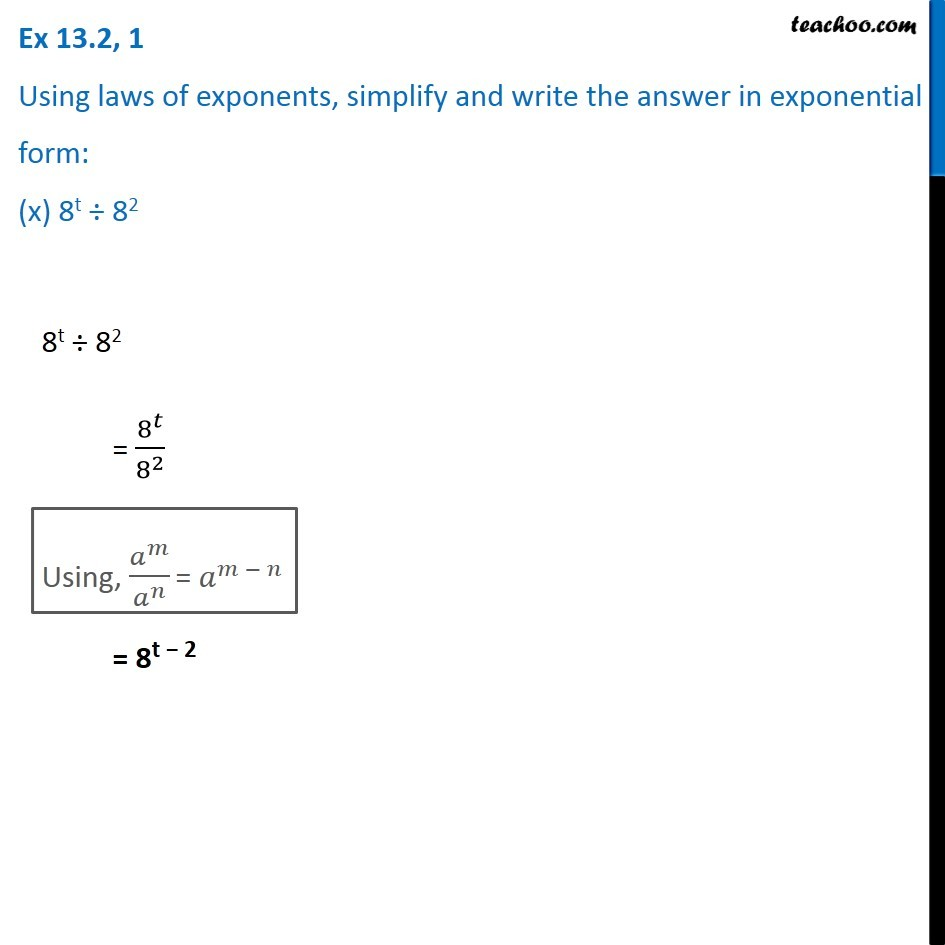1. Chapter 13 Class 7 Exponents and Powers
2. Serial order wise
3. Ex 13.2

Transcript

Ex 13.2, 1 Using laws of exponents, simplify and write the answer in exponential form: (i) 32 × 34 × 38 32 × 34 × 38 Using am × an = am + n = 32 + 4 + 8 = 36 + 8 = 314 Ex 13.2, 1 Using laws of exponents, simplify and write the answer in exponential form: (ii) 615 ÷ 610 615 ÷ 610 = 6^15/6^10 Using, 𝑎^𝑚/𝑎^𝑛 = 𝑎^(𝑚 − 𝑛) = 615 − 10 = 65 Ex 13.2, 1 Using laws of exponents, simplify and write the answer in exponential form: (iii) a3 × a2 a3 × a2 Using am × an = am + n = a3 + 2 = a5 Ex 13.2, 1 Using laws of exponents, simplify and write the answer in exponential form: (iv) 7𝑥 × 72 7𝑥 × 72 Using am × an = am + n = 7𝒙 + 2 Ex 13.2, 1 Using laws of exponents, simplify and write the answer in exponential form: (v) (5^2 )^3 ÷ 53 (5^2 )^3 ÷ 53 = 〖(52)〗^3/5^3 Using, (am)n = 𝑎^(𝑚 × 𝑛) Using, (am)n = 𝑎^(𝑚 × 𝑛) Using, 𝑎^𝑚/𝑎^𝑛 = 𝑎^(𝑚 − 𝑛) = 56 − 3 = 53

Ex 13.2